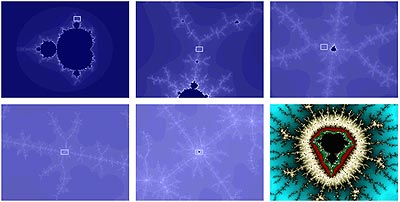QUESTION #458

# What are fractals?

A fractal is a mathematical object that is self-similar irrespective of scale.

So what does that mean? Well, the surface of a fractal has features on a large scale which are the same basic shape as smaller scale features, so when you look at a fractal and zoom in or out, it still basically looks the same.

A classic example is the Koch curve. Imagine an equilateral triangle. Then in the center third of each edge, place a smaller equilateral triangle. If you have it right you should now have a symmetrical six pointed star. Now in the central third of each edge, again place an equilateral triangle. (these edges are 1/9th the length of the original). Repeat an infinite number of times. As you zoom in on an edge, the shape looks the same forever. And what is the perimeter of this polygon you have just constructed? Infinity, even though it fits inside a circle with finite radius.We find fractals all the time in nature as well. Imagine the length of the coastline of England (to steal an example from James GleicK). How long is it? Imagine taking a stick one mile long and rolling it end over end along the coast and physically measuring the coast. You get some number of miles. But repeat the process with a stick only one meter long and you will get a much bigger distance (after you convert to miles, of course!) On a large scale, the big stick went through bays and estuaries, around peninsulae and promontories, but skipped over small dips and bumps. On a smaller scale, the one meter stick fits into smaller nooks and crannies and thus 'measures ' more of the coastline. Likewise a one centimeter stick will yield an even larger estimate of the length of the coastline as it wraps around individual pebbles and into tiny cracks. This physical analog to the Koch curve is not infinite, as there is a lower limit to the size of a stick due to atomic structure, but you get the idea.